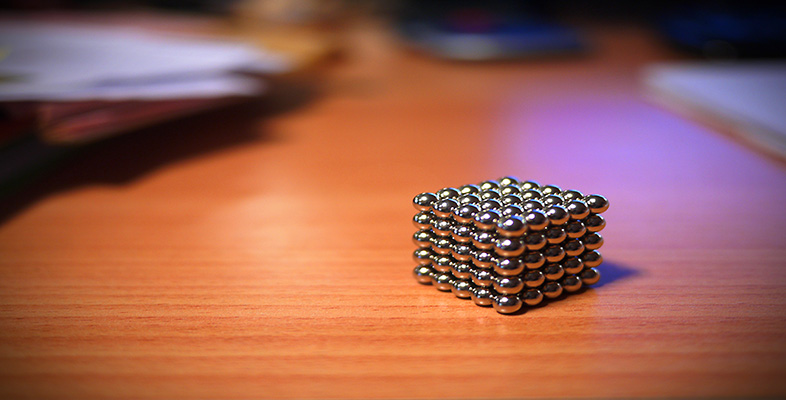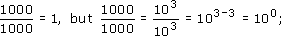Science, Maths & Technology

### Become an OU studentSquares, roots and powers

Start this free course now. Just create an account and sign in. Enrol and complete the course for a free statement of participation or digital badge if available.

# 2.5 The power zero

Now, what happens if you divide a number by itself? You should get the answer 1. For exampleso 100 must equal 1. This must be true for any number (except for 0, because there is a problem in defining 00). So, for example, 70 = 1, 1230 = 1, 10000 = 1. It even works for negative and decimal numbers. Check a few numbers on your calculator to see that raising to the power 0 gives 1.

Any non-zero number raised to the power 0 is 1.